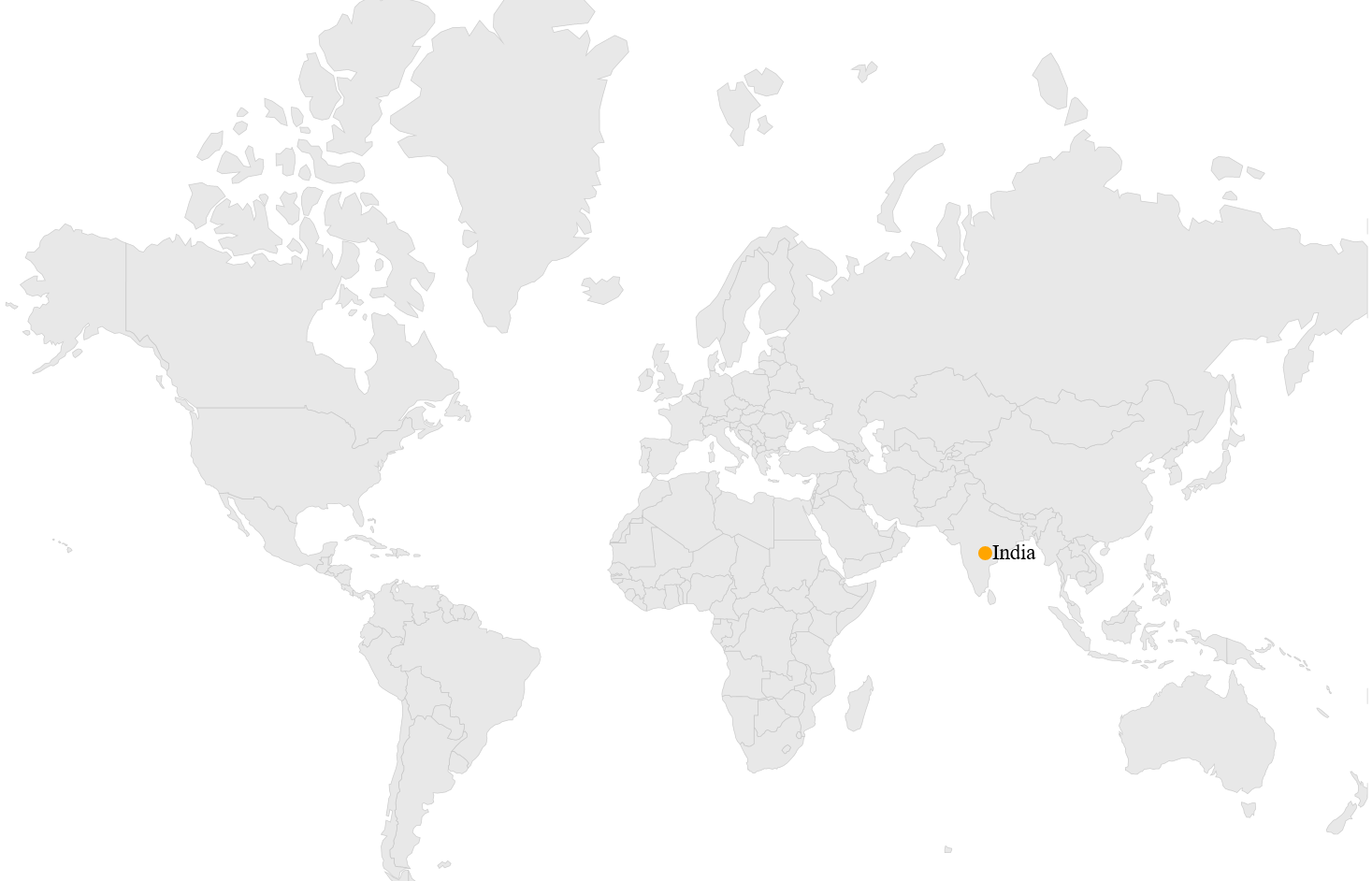# How to

## Transform latitude and longitude value to pixel value and vice-versa

SfMaps offers two utility methods to transform the pixel values to longitude and latitude values and vice-versa. This method is used for both ShapeFileLayer and ImageryLayer.

• `LatitudeLongitudeToPoint(double latitude, double longitude)` - Converts the latitude and longitude values to screen point. Here, pass the parameters as latitude and longitude values, from that values we can get screen points x and y.
• `GetLatLonFromPoint(Point point)` - Converts the screen point to longitude and latitude values. Here, pass the parameters as screen points x and y, from that points we can get longitude(Point.X) and latitude(Point.Y) values.
``````Point pixelPoint = layer.LatitudeLongitudeToPoint(21.00, 78.00);
Point longitudeLatitude = layer.GetLatLonFromPoint(pixelPoint);
mapAnnotations.Latitude = longitudeLatitude.Y;
mapAnnotations.Longitude = longitudeLatitude.X;``````